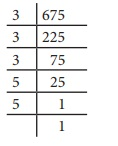Home | | Maths 8th Std | Cubes and Cube Roots

# Cubes and Cube Roots

If you multiply a number by itself and then by itself again, the result is a cube number.

Cubes and Cube Roots

If you multiply a number by itself and then by itself again, the result is a cube number.

This means that a cube number is a number that is the product of three identical numbers.

If n is a number, its cube is represented by n3.

Cube numbers can be represented visually as 3D cubes comprising of single unit cubes. Cube numbers are also called as perfect cubes. The perfect cubes of natural numbers are 1, 8, 27, 64, 125, 216, ... and so on.Ramanujan Number - 1729 = 123+13 = 103+93

Once Professor Hardy went to see Ramanujan when he was ill at Putney, riding in taxi cab number 1729 and said that the number seemed a dull one, and hoped it was not an unfavourable omen. “No,” replied Ramanujan and he completed saying “It is a very interesting number. Infact, it is the smallest number expressible as the sum of two cubes in two different ways.” 4104, 13832, 20683 are a few more examples of Ramanujan-Hardy numbers.

1. Properties of cubes of numbersNote

A perfect cube does not end with two zeroes.

The cube of a two digit number may have 4 or 5 or 6 digits in it.

Try these

Find the ones digit in the cubes of each of the following numbers.

1. 12

2. 27

3. 38

4. 53

5. 71

6. 84

Solution:

(i) 12

12 ends with 2, so its cube ends with 8 i.e, ones digit in 123 is 8.

(2) 27

27 ends with 7, so its cube end with 3. i.e., ones digit in 273 is 3.

(3) 38

38 ends with 8, so its cube ends with 2 i.e, ones digit in 383 is 2.

(4) 53

53 ends with 3, so its cube ends with 7. i.e, ones digit in 533 is 7.

(5) 71

71 ends with 1, so its cube ends with 1. i.e, ones digit in 713 is 1

(6) 84

84 ends with 4, so its cube ends with 4. i.e, ones digit in 843 is 4.

2. Cube root

The cube root of a number is the value that when cubed gives the original number.

For example, the cube root of 27 is 3 because when 3 is cubed we get 27.

Notation:

The cube root of a number x is denoted as

3x (or) x1/3

Here are some more cubes and cube roots:

3√1 = 1 since 13 = 1, 3√8 = 2 since 23 = 8,

327 = 3 since 33 = 27, 364 = 4 since 43 = 64,

3125 = 5 since 53 = 125 and so on.Example 1.32

Is 400 a perfect cube?

Solution:

By prime factorisation, we have 400 = 2 × 2 × 2 × 2 × 5 × 5

There is only one triplet. To make further triplets, we will need two more 2’s and one more 5.

Therefore, 400 is not a perfect cube.

Example 1.33

Find the smallest number by which 675 must be multiplied to obtain a perfect cube.

Solution:We find that, 675 = 3 × 3 × 3 × 5 × 5 …………….(1)

Grouping the prime factors of 675 as triplets, we are left over with 5 × 5.

We need one more 5 to make it a perfect cube.

To make 675 a perfect cube, multiply both sides of (1) by 5.

675×5=3×3×3×5×5×5

3375=3×3×3×5×5×5

Now, 3375 is a perfect cube. Thus, the smallest required number to multiply 675 such that the new number perfect cube is 5.

Think

In this question, if the word ‘multiplied’ is replaced by the word ‘divided’, how will the solution vary?

3. Cube root of a given number by Prime Factorisation

Step 1: Resolve the given number into the product of prime factors.

Step 2: Make triplet groups of same primes.

Step 3: Choosing one from each triplet, find the product of primes to get the cube root.

Example 1.34

Find the cube root of 27000.

Solution:

By prime factorisation, we have 27000 = 2× 2 ×2× 3 × 3 × 3 × 5 × 5 ×5

∴ 3√27000 = 2×3×5 =30

Example 1.35Tags : Numbers | Chapter 1 | 8th Maths , 8th Maths : Chapter 1 : Numbers
Study Material, Lecturing Notes, Assignment, Reference, Wiki description explanation, brief detail
8th Maths : Chapter 1 : Numbers : Cubes and Cube Roots | Numbers | Chapter 1 | 8th Maths